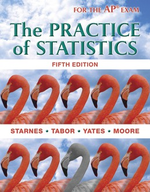### Solution Found!# Tall girls According to the National Center for Health Statistics, the distribution of

Chapter 7, Problem 10

(choose chapter or problem)

Get Unlimited Answers! Check out our subscriptions
QUESTION:

According to the National Center for Health Statistics, the distribution of heights for 16-year-old females is modeled well by a Normal density curve with mean $$\mu=64 \text { inches }$$ and standard deviation $$\sigma=2.5 \text { inches }$$. To see if this distribution applies at their high school, an AP® Statistics class takes an SRS of 20 of the 300 16-year-old females at the school and measures their heights. What values of the sample mean $$\bar{x}$$ would be consistent with the population distribution being N(64, 2.5)? To find out, we used Fathom software to simulate choosing 250 SRSs of size n = 20 students from a population that is N(64, 2.5). The figure below is a dotplot of the sample mean height $$\bar{x}$$ of the students in each sample.(a) There is one dot on the graph at 62.4. Explain what this value represents.

(b) Describe the distribution. Are there any obvious outliers?

(c) Would it be surprising to get a sample mean of 64.7 or more in an SRS of size 20 when $$\mu=64$$? Justify your answer.

(d) Suppose that the average height of the 20 girls in the class’s actual sample is $$\bar{x}=64.7$$. What would you conclude about the population mean height $$\mu$$ for the 16-year-old females at the school? Explain.

QUESTION:

According to the National Center for Health Statistics, the distribution of heights for 16-year-old females is modeled well by a Normal density curve with mean $$\mu=64 \text { inches }$$ and standard deviation $$\sigma=2.5 \text { inches }$$. To see if this distribution applies at their high school, an AP® Statistics class takes an SRS of 20 of the 300 16-year-old females at the school and measures their heights. What values of the sample mean $$\bar{x}$$ would be consistent with the population distribution being N(64, 2.5)? To find out, we used Fathom software to simulate choosing 250 SRSs of size n = 20 students from a population that is N(64, 2.5). The figure below is a dotplot of the sample mean height $$\bar{x}$$ of the students in each sample.(a) There is one dot on the graph at 62.4. Explain what this value represents.

(b) Describe the distribution. Are there any obvious outliers?

(c) Would it be surprising to get a sample mean of 64.7 or more in an SRS of size 20 when $$\mu=64$$? Justify your answer.

(d) Suppose that the average height of the 20 girls in the class’s actual sample is $$\bar{x}=64.7$$. What would you conclude about the population mean height $$\mu$$ for the 16-year-old females at the school? Explain.

Step 1 of 6

Given,

No. of SRS = 250

Sample size of an SRS, $$n=20$$

The mean, $$\mu=64 \text { inches }$$

The standard deviation, $$\sigma=2.5 \text { inches }$$